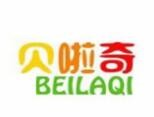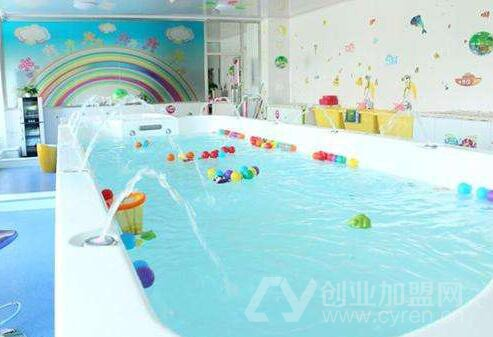|

• 餐饮
• 服装
• 家居
• 家纺
• 建材
• 美容
• 教育
• 干洗
• 保健
• 其他

# 加盟一个婴儿游泳馆需要多少钱？来看看详细费用分析！1租金费用

2设备费用

①豪华档：46000*1+2500*3+2000*2+2000*1+10000*1=69500元

②高档：32000*1+2000*3+1500*2+1500*1+8000*1=50500元

③中高档：30000*1+1600*3+1200*2+1500*1+8000*1=46700元

④中档：27000*1+1200*3+800*2+1500*1+8000*1=41700元

⑤普通档：24000*1+1000*3+600*2+1500*1+8000*1=37700元

3其他设备

4加盟费用

5装修费用

6配件耗材

• 项目很好，请尽快联系我详谈。
• 请问我所在的地区有加盟商了吗？
• 我想详细的了解加盟流程，请联系我！
• 留下邮箱，请将详细资料邮件给我
• 代理/加盟与你相伴能得到哪些支持？